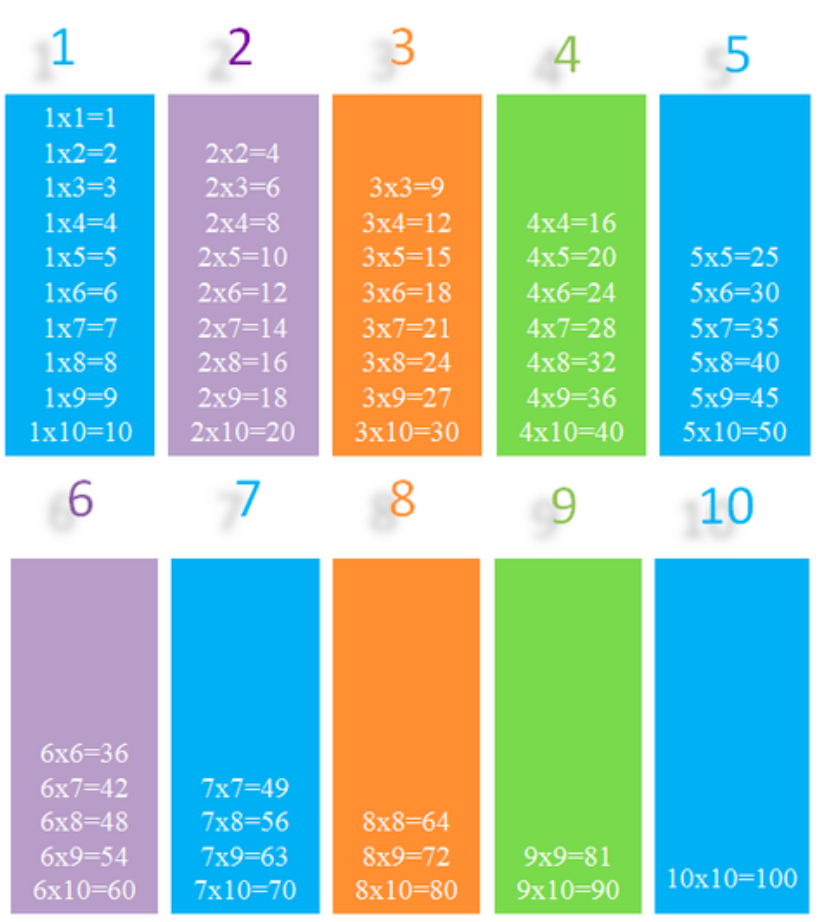Smartick is an online platform for children to master math in only 15 minutes a day

Aug18

# Learn the Different Properties of Multiplication

In this post, we will do a review of the properties of multiplication and look at some of its applications. We begin with a brief summary of the properties of multiplication:

### Properties of Multiplication

• Commutative Property: The order of the factors does not change the product.

3 x 5 = 5 x 3

• Associative Property: the grouping of factors does not vary the result of the multiplication.

(2 x 9) x 5 = 2 x (9 x 5)

• Neutral Element: 1 is the neutral element of multiplication because any number multiplied by 1 gives the same number.

5 x 1 = 5

154 x 1 = 154

• Distributive Property: The multiplication of the number by a sum is equal to the sum of the multiplication of said numbers by each summand.

3 x ( 5 + 2) = 3 x 5 + 3 x 2

Children often use the properties of multiplication without getting to understand them properly and without really knowing why they work.

### Through these examples we will try to understand it better:

We asked Carlos to add 60 + 30 and he answered, “60 plus 30 is 90 because 6 plus 3 is 9 and adding a zero to the end gives me 90.”

Many students learn to do this “trick” and it is correct, but do you know why it works? Symbolically, we can express it as follows:

60 + 30 =

Distributive Property:  (6 x 10) + (3 x 10) =

(6 + 3) x 10 =

9 x 10 = 90

One of the properties of multiplication, the distributive property, is implicit in the calculation, did you notice?

Now let’s look at another example: asking brothers Charles, Aaron, and Hugo, how much 8 x 6 is.

Aaron did not remember that 8 x 6 was 48, but knew it was like 8 x 5 plus 8. So he did “8 times 5 equals 40, I add 8 to 40 and that gives me 48. So 8 times 6 equals 48.” Aaron is right, 8 times 6 is 48, right?

We can express it as follows:

8 x 6=

Distributive Property:  8 x (5 + 1)=

8 x 5 + (8 x 1)=

8 x 5 + 8 =

40 + 8 = 48

And here we see again the distributive property of multiplication implicitly.
However, Hugo has reached the same solution but differently.

“I do not remember how much 8 x 6 is because I do not know the multiplication table for 8 yet, but I do know the multiplication table for 6 and 6 x 8 is 48 therefore 8 x 6 is 48.”

Has anyone noticed what property of multiplication he used? Indeed, Hugo has used the commutative property. Excellent!

To know and fully understand the properties of numbers and operations helps! Learning these is more efficient and provides different and more flexible ways to apply what we have learned in other fields such as algebra, geometry or problem-solving.

### Applications of the multiplication properties

When do we apply the distributive property? Among all the applications, we propose two cases:

• To make the multiplication of large numbers easier.

Example 1

102 x 5 = (100 + 2) x 5 = 100 x 5 + 2 x 5 = 500 + 10 = 510

Example 2

225 x 2 = (200 + 25) x 2 = 200 x 2 + 25 x 2 = 400 + 50 = 450

• To solve equations.

Example 3

3 x (5 + A) = 45

3 x 5 + 3 x A = 45

15 + 3 x A = 45

3 x A = 45-15

3 x A = 30

A = 10

When do we apply the associative property?

• To multiply more than two numbers more easily.

Example 4

(4 x 15) x 2 = 4 x (15 x 2) = 4 x 30 = 120

Finally, when do we apply the commutative property?

• When we learn the multiplication tables.

Knowing that the order of the factors does not change the product, you do not need to learn entire tables, look at these simplified multiplication tables:If you want to review all of the posts in which we have explained the distributive, associative and commutative properties check below:

In Smartick you have a lot of exercises where you can have fun learning math!